# dsp.ColoredNoise

Generate colored noise signal

## Description

The `dsp.ColoredNoise` System object™ generates a colored noise signal with a power spectral density (PSD) of 1/|f|α over its entire frequency range. The inverse frequency power, α, can be any value in the interval `[-2 2]`. The type of colored noise the object generates depends on the Color you choose. When you set `Color` to `'custom'`, you can specify the power density of the noise through the InverseFrequencyPower property.

The size and data type properties of the generated signal depend on SamplesPerFrame, NumChannels, and the OutputDataType properties.

This object uses the default MATLAB® random stream, `RandStream`. Reset the default stream for repeatable simulations.

To generate colored noise signal:

1. Create the `dsp.ColoredNoise` object and set its properties.

2. Call the object with arguments, as if it were a function.

## Creation

### Syntax

``cn = dsp.ColoredNoise``
``cn = dsp.ColoredNoise(Name,Value)``
``cn = dsp.ColoredNoise(pow,samp,numChan,Name,Value)``
``cn = dsp.ColoredNoise(color,samp,numChan,Name,Value)``

### Description

example

````cn = dsp.ColoredNoise` creates a colored noise object, `cn`, that outputs a noise signal one sample or frame at a time, with a 1/|f|α spectral characteristic over its entire frequency range. Typical values for α are α = 1 (pink noise) and α = 2 (brownian noise).```

example

````cn = dsp.ColoredNoise(Name,Value)` creates a colored noise object with each specified property set to the specified value. Enclose each property name in single quotes. Example: `dsp.ColoredNoise('Color','pink');````

example

````cn = dsp.ColoredNoise(pow,samp,numChan,Name,Value)` creates a colored noise object with the `InverseFrequencyPower` property set to pow, the `SamplesPerFrame` property set to samp, and the `NumChannels` property set to numChan. Example: `dsp.ColoredNoise(1,44.1e3,1,'OutputDataType','single');````

example

````cn = dsp.ColoredNoise(color,samp,numChan,Name,Value)` creates a colored noise object with the `Color` property set to color, the `SamplesPerFrame` property set to samp, and the `NumChannels` property set to numChan. Example: `dsp.ColoredNoise('pink',1024,2,'OutputDataType','single');````

## Properties

expand all

Unless otherwise indicated, properties are nontunable, which means you cannot change their values after calling the object. Objects lock when you call them, and the `release` function unlocks them.

If a property is tunable, you can change its value at any time.

Noise color, specified as one of the following. Each color is associated with a specific inverse frequency power of the generated noise sequence.

• `'pink'` –– The inverse frequency power, α equals 1.

• `'white'` –– α = 0.

• `'brown'` –– α = 2. Also known as red or Brownian noise.

• `'blue'` –– α = -1. Also known as azure noise.

• `'purple'` –– α = -2. Also known as violet noise.

• `'custom'` –– For noise with a custom inverse frequency power, α equals the value of the InverseFrequencyPower property.

`InverseFrequencyPower`, α can be any value in the interval [`-2,2`].

Inverse frequency power, α, specified as a real scalar in the interval `[-2 2]`. The inverse exponent defines the PSD of the random process as 1/|f|α. Values of InverseFrequencyPower greater than 0 generate lowpass noise with a singularity (pole) at f = 0. These processes exhibit long memory. Values of `InverseFrequencyPower` less than 0 generate highpass noise with increments that are negatively correlated. These processes are referred to as anti-persistent. Special cases include:

• `1` –– Pink noise

• `2` –– Brown, red, or Brownian noise

• `0` –– White noise process with a flat PSD

• `-1` –– Blue or azure noise

• `-2` –– Purple of violet noise

In a log-log plot of power as a function of frequency, processes generated by this object exhibit an approximate linear relationship with slope equal to –α.

Example: `1.2`

Example: `-1.4`

#### Dependencies

This property applies only when you set Color to `'custom'`.

Number of samples per output channel, specified as a positive integer. This property determines the number of rows of the signal.

Example: `512`

Number of output channels, specified as an integer. This property determines the number of columns of the signal.

Example: 5

Example: 25

Source of the random number stream, specified as one of the following:

• `'Global stream'` –– The current global random number stream is used for normally distributed random number generation.

• `'mt19937ar with seed'` –– The mt19937ar algorithm is used for normally distributed random number generation. The `reset` function reinitializes the random number stream to the value of the `Seed` property.

Initial seed of mt19937ar random number stream generator algorithm, specified as a nonnegative integer. The `reset` function reinitializes the random number stream to the value of the `Seed` property.

Example: `3`

Example: `34`

#### Dependencies

This property applies only when you set the `RandomStream` property to `'mt19937ar with seed'`.

Data Types: `double`

Specify the output to be bounded between +1 and −1, specified as:

• `true` –– The internal random source that generates the noise is uniform. If `Color` is set to `'white'`, there is no color filter applied to the output of the random source. The output is uniform noise of amplitude between +1 and −1. If `Color` is set to any other option, then a coloring filter is applied to the output of the random source, followed by a gain ensures that the absolute maximum output never exceeds `1`.

• `false` –– The internal random source is Gaussian. The output is not bounded.

Data Types: `logical`

Output data type, specified as either `'double'` or `'single'`.

## Usage

### Syntax

``noiseOut = cn()``

### Description

example

````noiseOut = cn()` outputs one sample or one frame of colored noise data.```

### Output Arguments

expand all

Colored noise output, returned as a vector or matrix. The SamplesPerFrame, NumChannels, and the OutputDataType properties specify the size and data type of the output.

Example: [`0.5377;2.1027;-1.1403;0.5885;0.6229;-0.8971;-0.7435;-0.0588;3.458;4.4537`]

## Object Functions

To use an object function, specify the System object as the first input argument. For example, to release system resources of a System object named `obj`, use this syntax:

`release(obj)`

expand all

 `step` Run System object algorithm `release` Release resources and allow changes to System object property values and input characteristics `reset` Reset internal states of System object

## Examples

collapse all

Note: If you are using R2016a or an earlier release, replace each call to the object with the equivalent `step` syntax. For example, `obj(x)` becomes `step(obj,x)`.

The output from this example shows that pink noise has approximately equal power in octave bands.

Generate a single-channel signal of pink noise that is 44,100 samples in length. Set the random number generator to the default settings for reproducible results.

```pinkNoise = dsp.ColoredNoise(1,44.1e3,1); rng default; x = pinkNoise();```

Set the sampling frequency to 44.1 kHz. Measure the power in octave bands beginning with 100-200 Hz and ending with 6.400-12.8 kHz. Display the results in a table.

```beginfreq = 100; endfreq = 200; count = 1; freqinterval = zeros(7,2); Pwr = zeros(7,1); while(endfreq<=44.1e3/2) freqinterval(count,:) = [beginfreq endfreq]; Pwr(count) = bandpower(x,44.1e3,[beginfreq endfreq]); beginfreq = endfreq; endfreq = 2*endfreq; count = count+1; end Pwr = Pwr(:); table(freqinterval,Pwr)```
```ans=7×2 table freqinterval Pwr _____________ _______ 100 200 0.17549 200 400 0.20313 400 800 0.2438 800 1600 0.2503 1600 3200 0.25233 3200 6400 0.26828 6400 12800 0.25211 ```

The pink noise has roughly equal power in octave bands.

Rerun the preceding code with `'InverseFrequencyPower'` equal to 0, which generates a white noise signal. A white noise signal has a flat power spectral density, or equal power per unit frequency. Set the random number generator to the default settings for reproducible results.

```whiteNoise = dsp.ColoredNoise(0,44.1e3,1); rng default; x = whiteNoise();```

Set the sampling frequency is 44.1 kHz. Measure the power in octave bands beginning with 100-200 Hz and ending with 6.400-12.8 kHz. Display the results in a table.

```beginfreq = 100; endfreq = 200; count = 1; while(endfreq<=44.1e3/2) freqinterval(count,:) = [beginfreq endfreq]; Pwr(count) = bandpower(x,44.1e3,[beginfreq endfreq]); beginfreq = endfreq; endfreq = 2*endfreq; count = count+1; end Pwr = Pwr(:); table(freqinterval,Pwr)```
```ans=7×2 table freqinterval Pwr _____________ _________ 100 200 0.0031417 200 400 0.0073833 400 800 0.017421 800 1600 0.035926 1600 3200 0.071139 3200 6400 0.15183 6400 12800 0.28611 ```

White noise has approximately equal power per unit frequency, so octave bands have an unequal distribution of power. Because the width of an octave band increases with increasing frequency, the power per octave band increases for white noise.

Note: If you are using R2016a or an earlier release, replace each call to the object with the equivalent `step `syntax. For example, `obj(x) `becomes `step(obj,x)`.

Generate a pink noise signal 2048 samples in length. The sampling frequency is 1 Hz. Obtain an estimate of the power spectral density using Welch's overlapped segment averaging.

```cn = dsp.ColoredNoise('pink','SamplesPerFrame',2048); x = cn(); Fs = 1; [Pxx,F] = pwelch(x,hamming(128),[],[],Fs,'psd');```

Construct the theoretical PSD of the pink noise process.

`PSDPink = 1./F(2:end);`

Display the Welch PSD estimate of the noise along with the theoretical PSD on a log-log plot. Plot the frequency axis with a base-2 logarithmic scale to clearly show the octaves. Plot the PSD estimate in dB, $10{\mathrm{log}}_{10}$.

```plot(log2(F(2:end)),10*log10(Pxx(2:end))) hold on plot(log2(F(2:end)),10*log10(PSDPink),'r','linewidth',2) xlabel('log_2(Hz)') ylabel('dB') title('Pink Noise') grid on legend('PSD estimate','Theoretical pink noise PSD') hold off```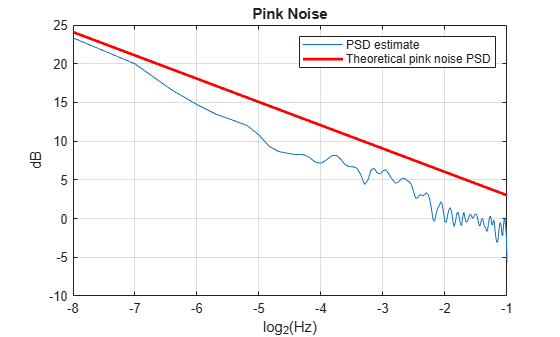Note: If you are using R2016a or an earlier release, replace each call to the object with the equivalent `step `syntax. For example, `obj(x) `becomes `step(obj,x)`.

Generate two channels of Brownian noise by setting `Color` to '`brown'` and `NumChannels` to 2.

```cn = dsp.ColoredNoise('brown','SamplesPerFrame',2048,... 'NumChannels',2); x = cn(); subplot(2,1,1) plot(x(:,1)); title('Channel 1'); axis tight; subplot(2,1,2) plot(x(:,2)); title('Channel 2'); axis tight;```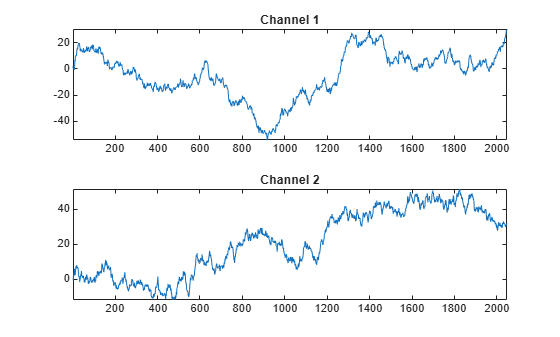The sampling frequency is 1 Hz. Obtain Welch PSD estimates for both channels. The fourth argument of `pwelch`, `NFFT`, which is the number of FFT points, is empty. Hence, `NFFT` is set to 256. For even NFFT, The number of FFT points used to calculate the PSD estimate is (NFFT/2+1), which equals 129.

```Fs = 1; Pxx = zeros(129,size(x,2)); for nn = 1:size(x,2) [Pxx(:,nn),F] = pwelch(x(:,nn),hamming(128),[],[],Fs,'psd'); end```

Construct the theoretical PSD of a Brownian process. Plot the theoretical PSD along with both realizations on a log-log plot. Use a base-2 logarithmic scale for the frequency axis and plot the power spectral densities in dB.

```PSDBrownian = 1./F(2:end).^2; figure; plot(log2(F(2:end)),10*log10(PSDBrownian),'k-.','linewidth',2); hold on; plot(log2(F(2:end)),10*log10(Pxx(2:end,:))); xlabel('log_2(Hz)'); ylabel('dB'); grid on; legend('Theoretical PSD','Channel 1', 'Channel 2');```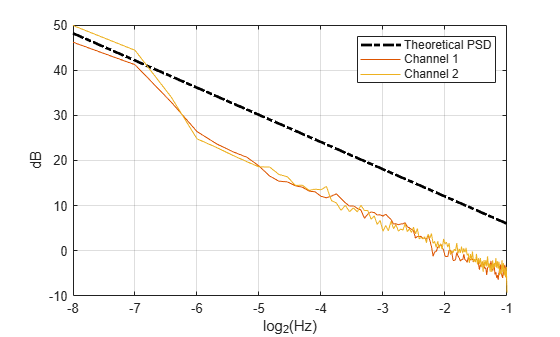Note: If you are using R2016a or an earlier release, replace each call to the object with the equivalent `step `syntax. For example, `obj(x) `becomes `step(obj,x)`.

Note: The `audioDeviceWriter` System object™ is not supported in MATLAB Online.

This example shows how to stream in an audio file and add pink noise at a 0 dB signal-to-noise ratio (SNR). The example reads in frames of an audio file 1024 samples in length, measures the root mean square (RMS) value of the audio frame, and adds pink noise with the same RMS value as the audio frame.

Set up the System objects. Set `'SamplesPerFrame'` for both the file reader and the colored noise generator to 1024 samples. Set `Color` to '`pink'` to generate pink noise with a $1/|f|$ power spectral density.

```N = 1024; afr = dsp.AudioFileReader('Filename','speech_dft.mp3','SamplesPerFrame',N); adw = audioDeviceWriter('SampleRate',afr.SampleRate); cn = dsp.ColoredNoise('pink','SamplesPerFrame',N);```

Stream the audio file in 1024 samples at a time. Measure the signal RMS value for each frame, generate a frame of pink noise equal in length, and scale the RMS value of the pink noise to match the signal. Add the scaled noise to the signal and play the output.

```while ~isDone(afr) audio = afr(); speechRMS = rms(audio); noise = cn(); noiseRMS = rms(noise); noise = noise*(speechRMS/noiseRMS); sigPlusNoise = audio+noise; adw(sigPlusNoise); end release(afr); release(adw);```

Note: If you are using R2016a or an earlier release, replace each call to the object with the equivalent `step` syntax. For example, `obj(x)` becomes `step(obj,x)`.

Generate two-channels of pink noise and compute the power spectrum based on a running average of 50 PSD estimates.

Set up the colored noise generator to generate two channels of pink noise with 1024 samples. Set up the spectrum analyzer to compute modified periodograms using a Hamming window and 50% overlap. Obtain a running average of the PSD using 50 spectral averages.

```pinkNoise = dsp.ColoredNoise('pink',1024,2); sa = dsp.SpectrumAnalyzer('SpectrumType','Power density', ... 'OverlapPercent',50,'Window','Hamming', ... 'SpectralAverages',50,'PlotAsTwoSidedSpectrum',false, ... 'FrequencyScale','log','YLimits',[-50 30]); ```

Run the simulation for 30 seconds.

```tic while toc < 30 pink = pinkNoise(); sa(pink); end ```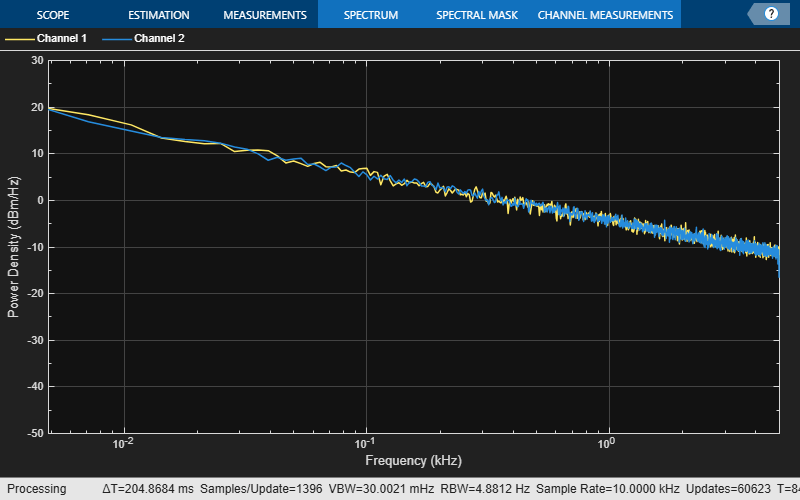expand all

## Algorithms

The figure shows the overall process of generating the colored noise.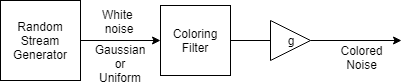The random stream generator produces a stream of white noise that is either Gaussian or uniform in distribution. A coloring filter applied to the white noise generates colored noise with a power spectral density (PSD) function given by:

`$S\left(f\right)=\frac{L\left(f\right)}{|f{|}^{\alpha }}$`

When α, the inverse frequency power, equals 0, no coloring filter is applied to the output of the random stream generator. If the bounded option is enabled, the output is uniform white noise with amplitude between +1 and −1. If the bounded output is not enabled, the output is a Gaussian white noise and the values are not bounded between +1 and −1. If α is set to any other value, then a coloring filter is applied to the output of the random stream generator. If the bounded output option is enabled, a gain g is applied to the output of the coloring filter to ensure that the absolute maximum output never exceeds `1`.

For details on colored noise processes and how the value of α affects the PSD of the colored noise, see Colored Noise Processes.

When the inverse frequency power α is positive, the colored noise is generated using an auto regressive (AR) model of order 63. The AR coefficients are:

`$\begin{array}{l}{a}_{0}=1,\\ {a}_{k}=\left(k-1-\frac{\alpha }{2}\right)\frac{{a}_{k-1}}{k},\text{\hspace{0.17em}}\text{\hspace{0.17em}}\text{\hspace{0.17em}}k=1,2,\dots ,63\end{array}$`

Pink and brown noises are special cases, which are generated from specially tuned SOS filters of orders 12 and 10, respectively. These filters are optimized for better performance.

When the inverse frequency power α is negative, the colored noise is generated using a moving average (MA) model of order 255. The MA coefficients are:

`$\begin{array}{l}{b}_{0}=1,\\ {b}_{k}=\left(k-1+\frac{\alpha }{2}\right)\frac{{b}_{k-1}}{k},\text{\hspace{0.17em}}\text{\hspace{0.17em}}k=1,2,\cdots ,255\end{array}$`

Purple noise is generated from a first order filter, B = [1 −1].

The coloring filters applied (except pink, brown, and purple) are detailed on pp. 820–822 in .

 Beran, J., Y. Feng, S. Ghosh, and R. Kulik, Long-Memory Processes: Probabilistic Properties and Statistical Methods. NewYork: Springer, 2013.

 Kasdin, N.J. "Discrete Simulation of Colored Noise and Stochastic Processes and 1/fα Power Law Noise Generation." Proceedings of the IEEE®, Vol. 83, No. 5, 1995, pp. 802–827.

## SupportGet trial now